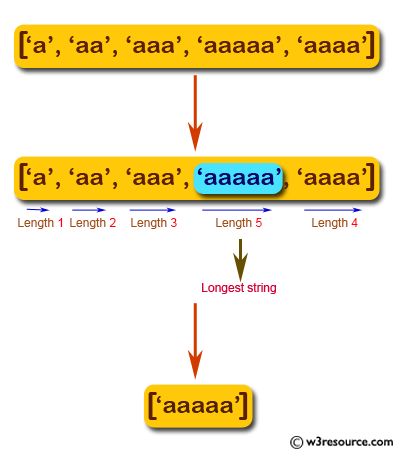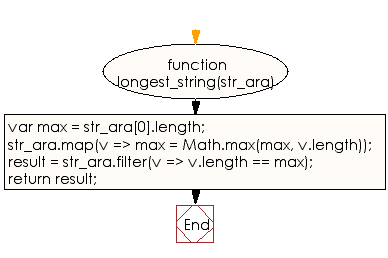# JavaScript: Find the longest string from a given array of strings

## JavaScript Basic: Exercise-83 with Solution

Write a JavaScript to find the longest string from a given array of strings.

Pictorial Presentation:Sample Solution:

HTML Code:

``````<!DOCTYPE html>
<html>
<meta charset="utf-8">
<meta name="viewport" content="width=device-width">
<title>JavaScript to find the longest string from a given array of strings</title>
<body>

</body>
</html>
```
```

JavaScript Code:

``````function longest_string(str_ara) {
var max = str_ara.length;
str_ara.map(v => max = Math.max(max, v.length));
result = str_ara.filter(v => v.length == max);
return result;
}

console.log(longest_string(['a', 'aa', 'aaa',
'aaaaa','aaaa']))
```
```

Sample Output:

```["aaaaa"]
```

Flowchart:ES6 Version:

``````function longest_string(str_ara) {
let max = str_ara.length;
str_ara.map(v => max = Math.max(max, v.length));
result = str_ara.filter(v => v.length == max);
return result;
}

console.log(longest_string(['a', 'aa', 'aaa',
'aaaaa','aaaa']))
``````

Live Demo:

See the Pen javascript-basic-exercise-83 by w3resource (@w3resource) on CodePen.

What is the difficulty level of this exercise?

Test your Programming skills with w3resource's quiz.

﻿

## JavaScript: Tips of the Day

Returns the sum of the powers of all the numbers from start to end (both inclusive)

Example:

```const sumPower = (end, power = 2, start = 1) =>
Array(end + 1 - start)
.fill(0)
.map((x, i) => (i + start) ** power)
.reduce((a, b) => a + b, 0);
console.log(sumPower(10)); // 385
console.log(sumPower(10, 3)); // 3025
console.log(sumPower(10, 3, 5)); // 2925
```

Output:

```385
3025
2925
```